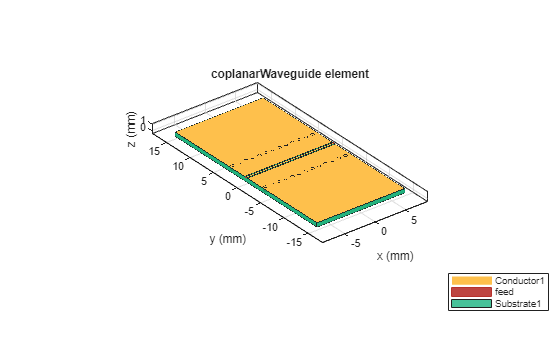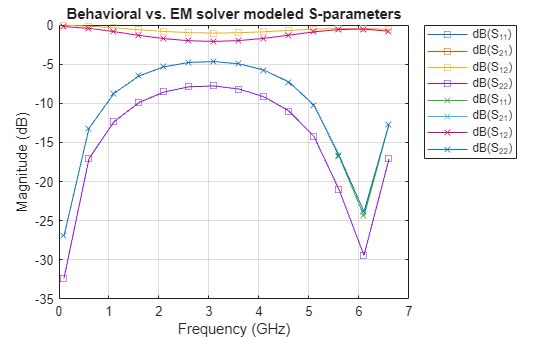txlineCPW

Create coplanar waveguide transmission line

Description

Use the txlineCPW object to create a coplanar waveguide (CPW) transmission line. You can also use the txlineCPW object to create an infinite-bottom conductor or ground plane CPW transmission line. The cross-section of a CPW transmission line is shown in this figure. The physical characteristics of the CPW transmission line include conductor width (w), conductor thickness (t), slot width (s), substrate height (d), and permittivity constant (ε).Creation

Description

txline = txlineCPW creates a default CPW transmission line object.

example

txline = txlineCPW(Name,Value) sets Properties using one or more name-value pairs. For example, txline = txlineCPW('SlotWidth',0.0046) creates a CPW transmission line with a slot width of 0.0046 meters.

Properties

expand all

Name of the CPW transmission line, specified as a string scalar or a character vector.

Example: 'Name','cpw1'

Example: txline.Name = 'cpw1'

Data Types: char | string

Physical width of the conductor, specified as a positive scalar in meters.

Example: 'ConductorWidth',0.0200

Example: txline.ConductorWidth = 0.0200

Data Types: double

Physical width of the slot, specified as a positive scalar in meters.

Example: 'SlotWidth',0.0008

Example: txline.SlotWidth = 0.0008

Data Types: double

Physical height of the conductor or dielectric thickness, specified as a positive scalar in meters.

Example: 'Height',0.000835

Example: txline.Height = 0.000835

Data Types: double

Physical thickness of the CPW transmission line, specified as a positive scalar in meters.

Example: 'Thickness',0.000008

Example: txline Thickness = 0.000008

Data Types: double

Relative permittivity of the dielectric, specified as a positive scalar.

Example: 'EpsilonR',8.8

Example: txline.EpsilonR = 8.8

Data Types: double

Loss angle tangent of the dielectric, specified as a nonnegative scalar.

Example: 'LossTangent',1

Example: txline.LossTangent = 1

Data Types: double

Conductivity of the conductor, specified as a nonnegative scalar in Siemens per meter (S/m).

Example: 'SigmaCond',2

Example: txline.SigmaCond = 2

Data Types: double

Physical length of the CPW transmission line, specified as a positive scalar in meters.

Example: 'LineLength',0.0200

Example: txline.LineLength = 0.0200

Data Types: double

Infinite-bottom conductor or ground plane, specified as a numeric or logical. When you specify 0, you create a transmission line without any conductor backing.

Example: 'ConductorBacked',1

Example: txline.ConductorBacked = 1

Data Types: double

Stub transmission line termination, specified as 'NotApplicable', 'Open', or 'Short'.

Example: 'Termination','Short'

Example: txline.Termination = 'Short'

Data Types: char

Type of stub, specified as 'NotAStub', 'Series', or 'Shunt'.

Example: 'StubMode','Series'

Example: txline.StubMode = 'Series'

Data Types: char

Number of input and output ports, returned as positive scalar.

Data Types: double

Terminals of the CPW transmission line, returned as a cell array of strings.

Data Types: char | string

Object Functions

 sparameters Calculate S-parameters for RF data, network, circuit, and matching network objects groupdelay Group delay of S-parameter object or RF filter object or RF Toolbox circuit object noisefigure Calculate noise figure of transmission lines, series RLC, and shunt RLC circuits getZ0 Calculate characteristic impedance with and without dispersion for transmission line circuit Circuit object clone Create copy of existing circuit element or circuit object

Examples

collapse all

Create a coplanar waveguide transmission line using these specifications:

• Conductor width : 45 mu m

• Slot width : 50 mu m

• Height of substrate : 525 mu m

• Thickness : 1 mu m

• GaAS permittivity or Epsilon R : 2.5 F/m

• Conductivity : 3.33e7 S/m

cpwtxline = txlineCPW('ConductorWidth',45e-6,'SlotWidth',50e-6,'Height',525e-6,...
'Thickness',1e-6,'EpsilonR',2.5,'SigmaCond',3.33e7)
cpwtxline =
txlineCPW: CPW element

Name: 'CPW'
ConductorWidth: 4.5000e-05
SlotWidth: 5.0000e-05
Height: 5.2500e-04
Thickness: 1.0000e-06
EpsilonR: 2.5000
LossTangent: 0
SigmaCond: 33300000
LineLength: 0.0100
Termination: 'NotApplicable'
StubMode: 'NotAStub'
NumPorts: 2
Terminals: {'p1+'  'p2+'  'p1-'  'p2-'}

Calculate the S-parameters of the transmission line at 20 GHz.

sparam = sparameters(cpwtxline,20e9);

This example uses RF PCB Toolbox to calculate an electromagnetic (EM) solver S-parameter model of a conductor-backed coplanar waveguide (CPW) transmission line.

Behavioral Modeling

Select the FR4 dielectric and silver metal the metal and dielectric libraries, respectively, of RF PCB Toolbox.

dFR4 = dielectric('FR4');
mSilver = metal('Silver');

Design an infinite-bottom conductor-backed CPW transmission line at 6 GHz and ${\mathit{Z}}_{0}$ of 75$\Omega$.

tx = txlineCPW('ConductorBacked',true, ...
'LineLength',0.0145,'ConductorWidth',2.6482e-4, ...
'SlotWidth',2e-4,'Height',6.35e-4, ...
"EpsilonR",dFR4.EpsilonR,"LossTangent",dFR4.LossTangent, ...
"SigmaCond",mSilver.Conductivity,'Thickness',2e-7, ...
'StubMode',"NotAStub","Termination","NotApplicable");

Calculate the S-parameters using a behavioral model from RF Toolbox with the reference impedance of 50$\Omega$. The behavioral model is also called an analytical or closed-form model.

freq = (1:5:66)*100e6;
Srf = sparameters(tx,freq,50);

EM Modeling

Create an RF PCB Toolbox coplanarWaveguide object.

tx_em = coplanarWaveguide(tx);

View the conductor-backed CPW transmission line.

show(tx_em)Calculate the S-parameters using the EM solver from RF PCB Toolbox.

Sem = sparameters(tx_em,freq,50);

Compare S-parameters

Compare the S-parameters from the behavioral and EM solver models.

rfplot(Srf,'-s','db')
hold on
rfplot(Sem,'-x','db')
title('Behavioral vs. EM solver modeled S-parameters')Compatibility Considerations

expand all

Behavior changed in R2021b

 Garg, Ramesh, I. J. Bahl, and Maurizio Bozzi. Microstrip Lines and Slotlines . 3rd ed. Artech House Microwave Library. Boston: Artech House, 2013.